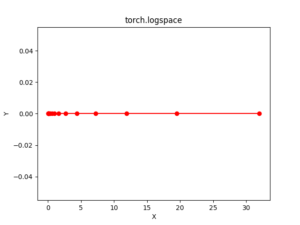Related Articles

# Python Pytorch logspace() method

• Last Updated : 22 Apr, 2020

PyTorch is an open-source machine learning library developed by Facebook. It is used for deep neural network and natural language processing purposes.

The function torch.logspace() returns a one-dimensional tensor of steps points logarithmically spaced with base base between.

The output tensor is 1-D of size steps.

Syntax: torch.logspace(start, end, steps=100, base=10, out=None)

Parameters:
start: the starting value for the set of point.
end: the ending value for the set of points
steps: number of points to sample between start and end. Default: 100.
base: base of the logarithm function. Default: 10.0
out(Tensor, optional): the output tensor

Return type: A tensor

Code #1:

 # Importing the PyTorch libraryimport torch  # Applying the logspace function and# storing the resulting tensor in 't'a = torch.logspace(3, 10, 5)print("a = ", a)  b = torch.logspace(start =-10, end = 10, steps = 5)print("b = ", b)

Output:

a =  tensor([1.0000e+03, 5.6234e+04, 3.1623e+06, 1.7783e+08, 1.0000e+10])
b =  tensor([1.0000e-10, 1.0000e-05, 1.0000e+00, 1.0000e+05, 1.0000e+10])


Code #2: Visualization

 # Importing the PyTorch libraryimport torch# Importing the NumPy libraryimport numpy as np  # Importing the matplotlib.pylot functionimport matplotlib.pyplot as plt  # Applying the logspace function to get a tensor of size 15 with values from -5 to 5 using base 2a = torch.logspace(-5, 5, 15, 2)print(a)  # Plottingplt.plot(a.numpy(), np.zeros(a.numpy().shape), color = 'red', marker = "o") plt.title("torch.linspace") plt.xlabel("X") plt.ylabel("Y")   plt.show()

Output:

tensor([3.1250e-02, 5.1271e-02, 8.4119e-02, 1.3801e-01, 2.2643e-01, 3.7150e-01,
6.0951e-01, 1.0000e+00, 1.6407e+00, 2.6918e+00, 4.4164e+00, 7.2458e+00,
1.1888e+01, 1.9504e+01, 3.2000e+01])
[torch.FloatTensor of size 15]Attention geek! Strengthen your foundations with the Python Programming Foundation Course and learn the basics.

To begin with, your interview preparations Enhance your Data Structures concepts with the Python DS Course. And to begin with your Machine Learning Journey, join the Machine Learning – Basic Level Course

My Personal Notes arrow_drop_up